# Texas Go Math Grade 3 Lesson 8.5 Answer Key Multiply with 9

Refer to our Texas Go Math Grade 3 Answer Key Pdf to score good marks in the exams. Test yourself by practicing the problems from Texas Go Math Grade 3 Lesson 8.5 Answer Key Multiply with 9.

## Texas Go Math Grade 3 Lesson 8.5 Answer Key Multiply with 9

Essential Question
What strategies can you use to multiply with 9?

Unlock the Problem
Lindsey’s class is studying the solar system. Seven students are making models of the solar system. Each model has 9 spheres (eight for the planets and one for Pluto, a dwarf planet). How many spheres do the 7 students need for all the models?

• What are you asked to find?
How many spheres do the 7 students need for all the models?

Explanation:
It is asked to find how many spheres do the 7 students need for all the models?

• How many students are making models?
7 students are making models.

Explanation:
Number of students making models = 7.

Find 7 × 9.
One Way
Use the Distributive Property.B. With multiplication and subtraction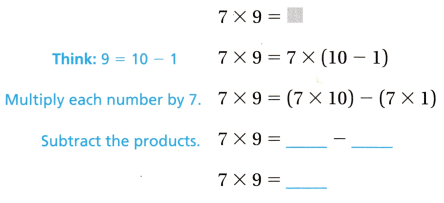So, 7 students need ___ spheres for all models.

Another Way
Use patterns of 9.
The table shows the 9s facts.

• What do you notice about the tens digit in the product?
The tens digit is ___ less than the factor that is multiplied by 9.
• What do you notice about the sum of the digits in the product?

The sum of the digits in the product is always ___.
So, to multiply 7 × 9, think: the tens digit is ___ and the ones digit is ___. The product is ___.Try This!
Complete the table above.
Use the patterns to find 8 × 9 and 9 × 9.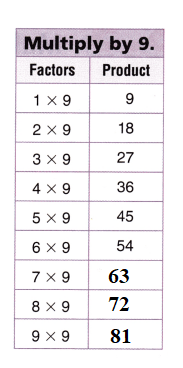Explanation:
7 × 9 = 7 × (3 + 6)
= (7 × 3) + (7 × 6)
= 21 + 42
= 63.
8 × 9 = 8 × (3 + 6)
= (8 × 3) + (8 × 6)
= 24 + 48
= 72.
9 × 9 = 9 × (3 + 6)
= (9 × 3) + (9 × 6)
= 27 + 54
= 81.

Math Talk
Mathematical Processes
Explain how you know the ones digit in the product 3 × 9.
When we have to calculate the unit digit of any function we need to work on the unit digit only i.e. all operations like addition,multiplication etc will be carried out on the unit digits.

Explanation:
You see, when we have to calculate the unit digit of any function we need to work on the unit digit only i.e. all operations like addition,multiplication etc will be carried out on the unit digits.
3 × 9 = 3 × (3 + 6)
= (3 × 3) + (3 × 6)
= 9 + 18
= 27.

Share and Show
Question 1.
What is the tens digit in the product 3 × 9? ___
Think: What number is 1 less than 3?
The tens digit in the product 3 × 9 is 2.

Explanation:
3 × 9 = 3 × (10 – 1)
= (3 × 10) – (3 × 1)
= 30 – 3
= 27.

Find the product.

Question 2.
9 × 8 = ___
9 × 8 = 72.

Explanation:
9 × 8 = (3 + 6) × 8
= (3 × 8) + (6 × 8)
= 24 + 48
= 72.

Question 3.
__ = 2 × 9
18 = 2 × 9.

Explanation:
2 × 9 = 2 × (3 + 6)
= (2 × 3) + (2 × 6)
= 6 + 12
= 18.

__ = 6 × 9
54 = 6 × 9.

Explanation:
6 × 9 = 6 × (3 + 6)
= (6 × 3) + (6 × 6)
= 18 + 36
= 54.

Question 5.
9 × 1 = __
9 × 1 = 9.

Explanation:
9 × 1 = (3 + 6) × 1
= (3 × 1) + (6 × 1)
= 3 + 6
= 9.

Question 6.
4 × 9 = __
4 × 9 = 36.

Explanation:
4 × 9 = 4 × (3 + 6)
= (4 × 3) + (4 × 6)
= 12 + 24
= 36.

Question 7.
5 × 9 = __
5 × 9 = 45.

Explanation:
5 × 9 = 5 × (3 + 6)
= (5 × 3) + (5 × 6)
= 15 + 30
= 45.

Question 8.
10 × 9 = __
10 × 9 = 90.

Explanation:
10 × 9 = 10 × (3 + 6)
= (10 × 3) + (10 × 6)
= 30 + 60
= 90.

Question 9.
9 × 9 = __
9 × 9 = 81.

Explanation:
9 × 9 = 9 × (3 + 6)
= (9 × 3) + (9 × 6)
= 27 + 54
= 81.

Problem Solving
H.O.T. Algebra Compare. Write <, >, or =.
Question 10.
2 × 9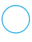3 × 6
2 × 9 = 3 × 6.

Explanation:
2 × 9 = 18.
3 × 6 = 18.

Question 11.
5 × 96 × 7
5 × 9  > 6 × 7.

Explanation:
5 × 9 = 45.
6 × 7 = 42.

Question 12.
1 × 93 × 3
1 × 9  = 3 × 3.

Explanation:
1 × 9 = 9.
3 × 3 = 9.

Question 13.
9 × 47 × 5
9 × 4  > 7 × 5.

Explanation:
9 × 4 = 36.
7 × 5 = 35.

9 × 02 × 3
9 × 0  <  2 × 3.

Explanation:
9 × 0 = 0.
2 × 3 = 6.

Question 15.
5 × 83 × 9
5 × 8  > 3 × 9.

Explanation:
5 × 8 = 40.
3 × 9 = 27.

Problem Solving
Use the table for 16-19.
Question 16.
The number of moons for one of the planets can be found by multiplying 7 × 9. Which planet is it?
Jupiter planet it is.

Explanation:
7 × 9 = 7 × (3 + 6)
= (7 × 3) + (7 × 6)
= 21 + 42
= 63.
Jupiter has 63 moons.

Question 17.
H.O.T. Uranus has 27 moons. What multiplication fact with 9 can be used to find the number of moons Uranus has? Use Math Language to describe how you can find the fact.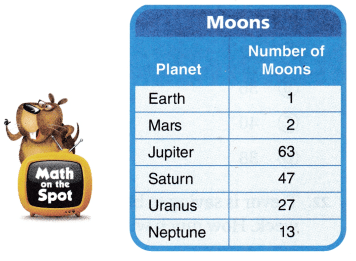3 × 9 = 27 multiplication fact with 9 can be used to find the number of moons Uranus has.

Explanation:
Uranus has 27 moons.
=> ?? × 9 = 27.
= ?? = 27 ÷ 9
= ?? = 3.

Question 18.
H.O.T. Multi-Step This planet has 9 times the number of moons that Mars and Earth have together. Which planet is it? Explain your answer.
Uranus has 27 moons.

Explanation:
Number of moons Mars has = 2.
Number of moons Earth has = 1.
Total number of moons Mars and Earth has = Number of moons Mars has + Number of moons Earth has
= 2 + 1
= 3.
This planet has 9 times the number of moons that Mars and Earth have together.
=> 3 × 9 = 3 × (3 + 6)
= (3 × 3) + (3 × 6)
= 9 + 18
= 27.

Question 19.
Write Math What’s the Question? Nine students made models of Mars and its moons. The answer is 18. What’s the question?
Question:
Nine students made models of Mars and its moons. The answer is 18. How many moons are there for Mars?

Explanation:
Nine students made models of Mars and its moons. The answer is 18. What’s the question?
Question:
Nine students made models of Mars and its moons. The answer is 18. How many moons are there for Mars?

Question 20.
Multi-Step The school library has 97 books about space. John and 3 of his friends each check out 9 books. How many space books are still in the school library?
Number of space books are still in the school library = 61.

Explanation:
Number of books about space the school library has = 97.
Number of books John and 3 of his friends each check out = 9.
Number of people took books check out = 4.
Number of space books are still in the school library = Number of books about space the school library has – (Number of books John and 3 of his friends each check out × Number of people took books check out)
= 97 – (9 × 4)
= 97 – 36
= 61.

Fill in the bubble for the correct answer choice.
Question 21.
A sea otter has 4 paws. How many paws do 9 sea otters have?
(A) 32
(B) 36
(C) 40
(D) 38
Number of paws paws 9 sea otters have = 36.
(B) 36.

Explanation:
Number of paws a sea otter has = 4.
Number of paws paws 9 sea otters have = 9 × Number of paws a sea otter has
= 9 × 4
= (3 + 6) × 4
= (3 × 4) + (6 × 4)
= 12 + 24
= 36.

Trevor is saving to buy new skates. He saves $10 each week. How much will he have saved after 9 weeks? (A)$80
(B) $100 (C)$19
(D) $90 Answer: Amount of money he have saved after 9 weeks =$90.
(D) $90. Explanation: Amount of money each week he saves =$10.
Amount of money he have saved after 9 weeks = 9 × $10 = (3 + 6) ×$10
= (3 × $10) + (6 ×$10)
= $30 +$60
= \$90.

Question 23.
Multi-Step There are 4 girls and 5 boys in Kara’s class. Each student in the class takes 2 books from the library. How many books does the class take from the library?
(A) 18
(B) 11
(C) 8
(D) 16
Number of books the class take from the library = 18.
(A) 18.

Explanation:
Number of boys in Kara’s class = 5.
Number of girls in Kara’s class = 4.
Number of books each students takes = 2.
Number of books the class take from the library = (Number of boys in Kara’s class + Number of girls in Kara’s class) × Number of books each students takes
= (5 + 4) × 2
= (5 × 2) + (4 × 2)
= 10 + 8
= 18.

Texas Test Prep
Question 24.
Joel has 5 shelves of model airplanes. He has 9 airplanes on each shelf. How many model airplanes does he have?
(A) 45
(B) 9
(C) 5
(D) 36
Number of model airplanes he have = 45.
(A) 45.

Explanation:
Number of shelves of model airplanes Joel has = 5.
Number of airplanes on each shelf he has = 9.
Number of model airplanes he have = Number of shelves of model airplanes Joel has × Number of airplanes on each shelf he has
= 5 × 9
= 5 × (3 + 6)
= (5 × 3) + (5 × 6)
= 15 + 30
= 45.

### Texas Go Math Grade 3 Lesson 8.5 Homework and Practice Answer Key

Find the product.

Question 1.
9 × 3 = ___
9 × 3 = 27.

Explanation:
9 × 3 = (3 + 6) × 3
= (3 × 3) + (6 × 3)
= 9 + 18
= 27.

Question 2.
___ = 9 × 6
54 = 9 × 6.

Explanation:
9 × 6 = (3 + 6) × 6
= (3 × 6) + (6 × 6)
= 18 + 36
= 54.

Question 3.
4 × 9 = ___
4 × 9 = 36.

Explanation:
4 × 9 = 4 × (3 + 6)
= (4 × 3) + ( 4 × 6)
= 12 + 24
= 36.

Question 4.
7 × 9 = ___
7 × 9 = 63.

Explanation:
7 × 9 = 7 × (3 + 6)
= (7 × 3) + (7 × 6)
= 21 + 42
= 63.

9 × 5 = ___
9 × 5 = 45.

Explanation:
9 × 5 = (3 + 6) × 5
= (3 × 5) + (6 × 5)
= 15 + 30
= 45.

Question 6.
__ = 9 × 9
81 = 9 × 9.

Explanation:
9 × 9 = 9 × (3 + 6)
= (9 × 3) + (9 × 6)
= 27 + 54
= 81.

Problem Solving
Use the table for 7-9.
Question 7.
The number of points scored for which team can be found by multiplying 6 × 9?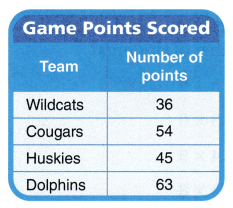Cougars team has the points of 6 × 9 = 54.

Explanation:-
6 × 9 = 6 × (3 + 6)
= (6 × 3) + ( 6 × 6)
= 18 + 36
= 54.

Question 8.
The Huskies scored 45 points. What multiplication fact with 9 can be used to find the number of points?
5 × 9 = 45 multiplication fact with 9 can be used to find the number of points.

Explanation:
The Huskies scored 45 points.
=> ?? × 9 = 45.
=> ?? = 45 ÷ 9
=> ?? = 5.

Question 9.
Suppose the Dolphins score another 9 points. What multiplication fact with 9 can be used to find the total points?
8  ×  9 = 72 multiplication fact with 9 can be used to find the total points.

Explanation:
Number of points Dolphins scored = 63.
Suppose the Dolphins score another 9 points.
=> Total number of points Dolphins scored = Number of points Dolphins scored + 9
= 63 + 9
= 72.
Multiplication fact with 9:
?? × 9 = 72
=> ?? = 72 ÷ 9
=> ?? = 8.

Question 10.
A school bus has 2 × 9 girls and 27 boys. How many girls are on the bus? What multiplication fact can be used to find the number of boys?
Number of girls school bus has = 18.
3 × 9 = 27 multiplication fact can be used to find the number of boys.

Explanation:
Number of girls school bus has = 2 × 9
= 9 + 9
= 18.
Number of boys school bus has = 27.
=> ?? × 9 = 27.
=> ?? = 27 ÷ 9
=> ?? = 3.

Question 11.
There are 4 students on each relay team in a race. There are 36 students in all. What multiplication fact can be used to show how many relay teams there are?
4 × 9 = 36 multiplication fact can be used to show how many relay teams there are.

Explanation:
Number of students on each relay team in a race = 4.
Total number of students in all = 36.
Number of relay teams there are  × Number of students on each relay team in a race = Total number of students in all
=> ?? × 4 = 36
=> ?? = 36 ÷ 4
=> ?? = 9.

Texas Test Prep
Lesson Check
Question 12.
An octopus has 8 arms. How many arms do 9 octopuses have?
(A) 81
(B) 63
(C) 89
(D) 72
Number of arms 9 octopuses have = 72.
(D) 72.

Explanation:
Number of arms an octopus has = 8.
Number of arms 9 octopuses have = 9 × Number of arms an octopus has
= 9 × 8
= (3 + 6) × 8
= (3 × 8) + (6 × 8)
= 24 + 48
= 72.

Question 13.
A starfish has 5 arms. How many arms do 9 starfish have?
(A) 45
(B) 50
(C) 35
(D) 59
Number of arms 9 starfish have = 45.
(A) 45.

Explanation:
Number of arms a starfish has = 5.
Number of arms 9 starfish have = 9 × Number of arms a starfish has
= 9 × 5
= (3 + 6) × 5
= (3 × 5) + (6 × 5)
= 15 + 30
= 45.

Question 14.
Miss Lincoln has a total of 27 students in her class. Which fact can be completed to show the number of students?
(A) 3 + 9
(B) 3 × 9
(C) 4 × 9
(D) 4 × 8
Multiplication fact showing number of students = 3 × 9.
(B) 3 × 9.

Explanation:
Total number of students in her class Miss Lincoln has = 27.
Multiplication fact showing number of students = ?? × 9 = 27
=> ?? = 27 ÷ 9
=> ?? = 3.

Question 15.
Deanna puts 63 postcards into groups of 7. Which number sentence shows the number of postcards?
(A) 8 × 7 = 63
(B) 7 + 9 = 63
(C) 8 × 9 = 63
(D) 7 × 9 = 63
Multiplication fact showing the number of postcards = 7 × 9 = 63.
(B) 7 + 9 = 63.

Explanation:
Number of postcards Deanna has = 63.
Number of groups he puts them = 7.
Multiplication fact of number of postcards:
Number of postcards Deanna has =  Number of groups he puts them × ??
=> 63 = 7 × ??
=> 63 ÷ 7 = ??
=> 9 = ??

Question 16.
Multi-Step There are 3 girls and 6 boys at a table in art class. Each student paints 3 pictures. How many pictures do the students paint in all?
(A) 12
(B) 27
(C) 18
(D) 21
Number of pictures the students paint in all = 27.
(B) 27.

Explanation:
Number of girls at a table in art class = 3.
Number of boys at a table in art class = 6.
Number of pictures each student paints = 3.
Number of pictures the students paint in all = (Number of girls at a table in art class + Number of boys at a table in art class) × Number of pictures each student paints
= (3 + 6) × 3
= (3 × 3) + (6 × 3)
= 9 + 18
= 27.

Question 17.
Multi-Step Frank makes a tile design with 4 rows of 9 tiles. If he adds 3 more rows to the tiles, how many tiles will he have in his design?
(A) 54
(B) 36
(C) 16
(D) 63
Number of tiles will he have in his design = 63.
(D) 63.

Explanation:
Number of rows of tiles = 4.
Number of tiles Frank makes a tile design = 9.
Number of more rows of tiles added = 3.
Number of tiles will he have in his design = (Number of rows of tiles + Number of more rows of tiles added) × Number of tiles Frank makes a tile design
= (4 + 3) × 9
= 7 × (3 + 6)
= (7 × 3) + (7 × 6)
= 21 + 42
= 63.

Scroll to Top